Tangents and Normals (using Differentiation)

Chapter 6 Class 12 Application of Derivatives
Serial order wise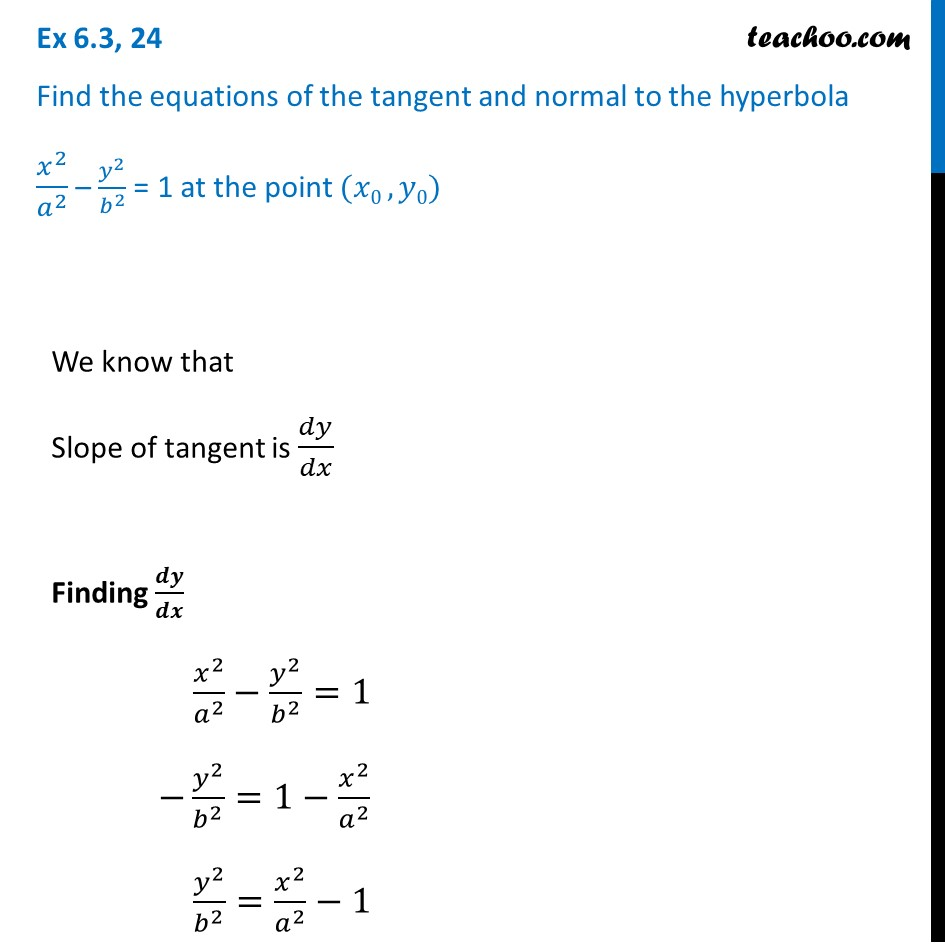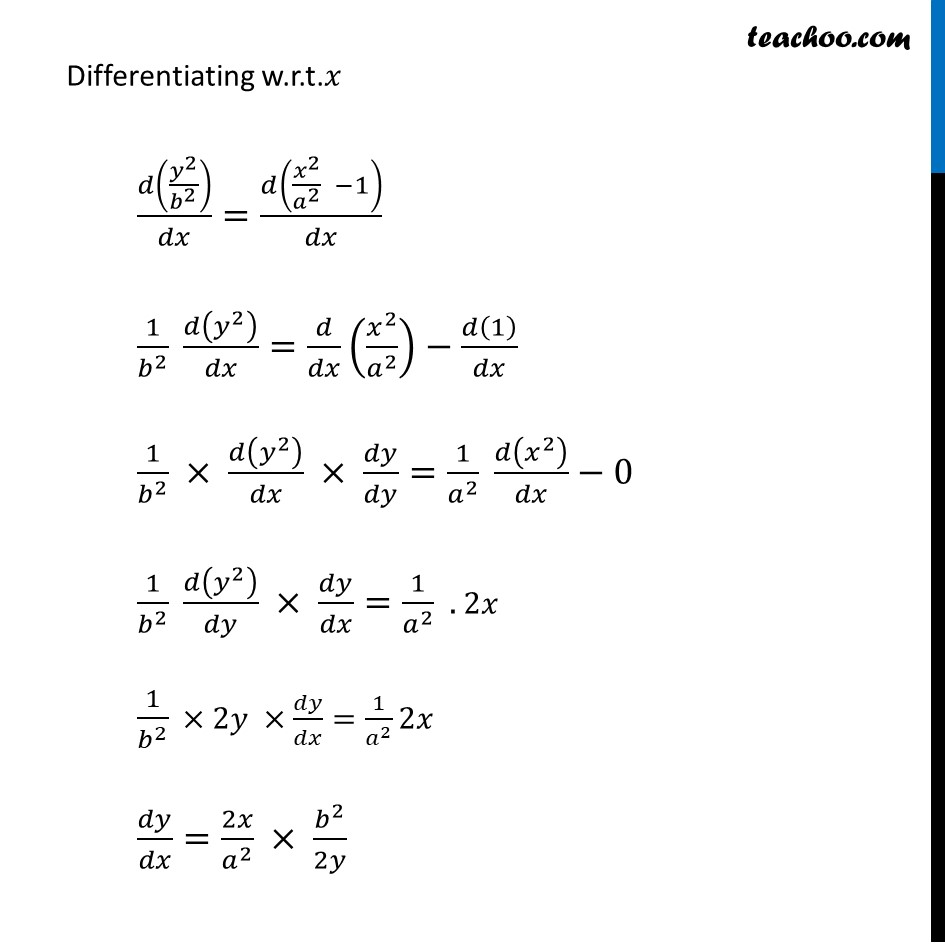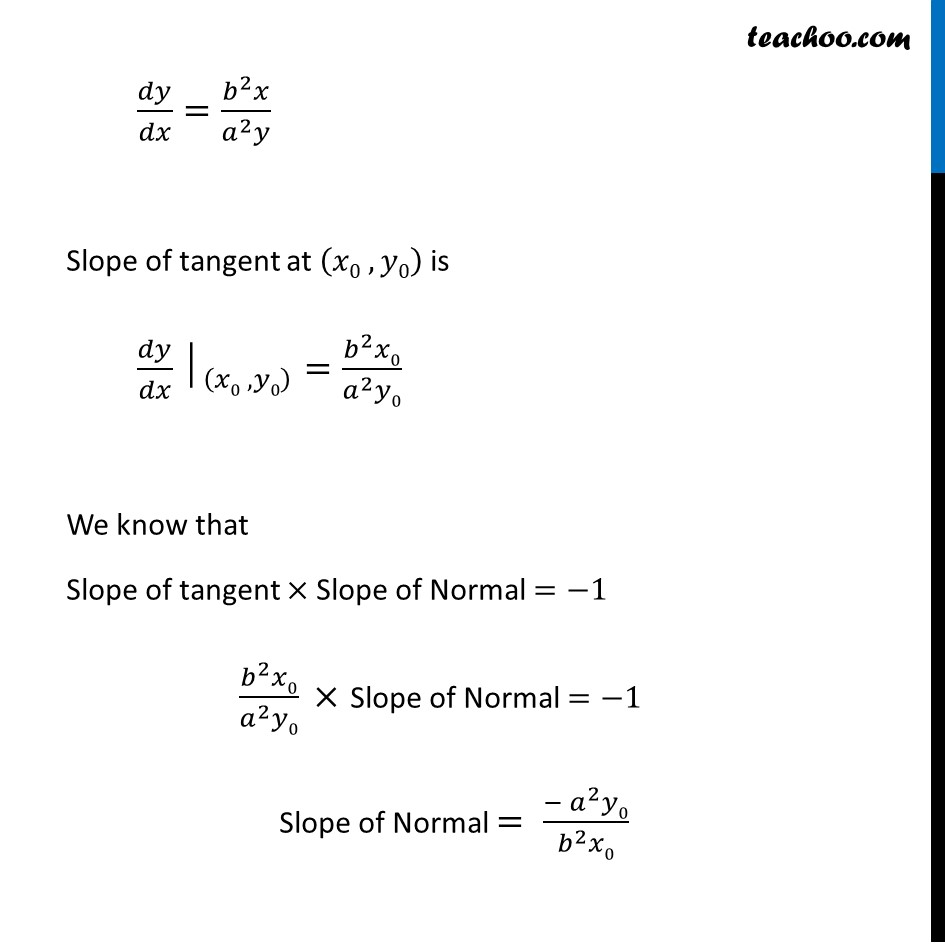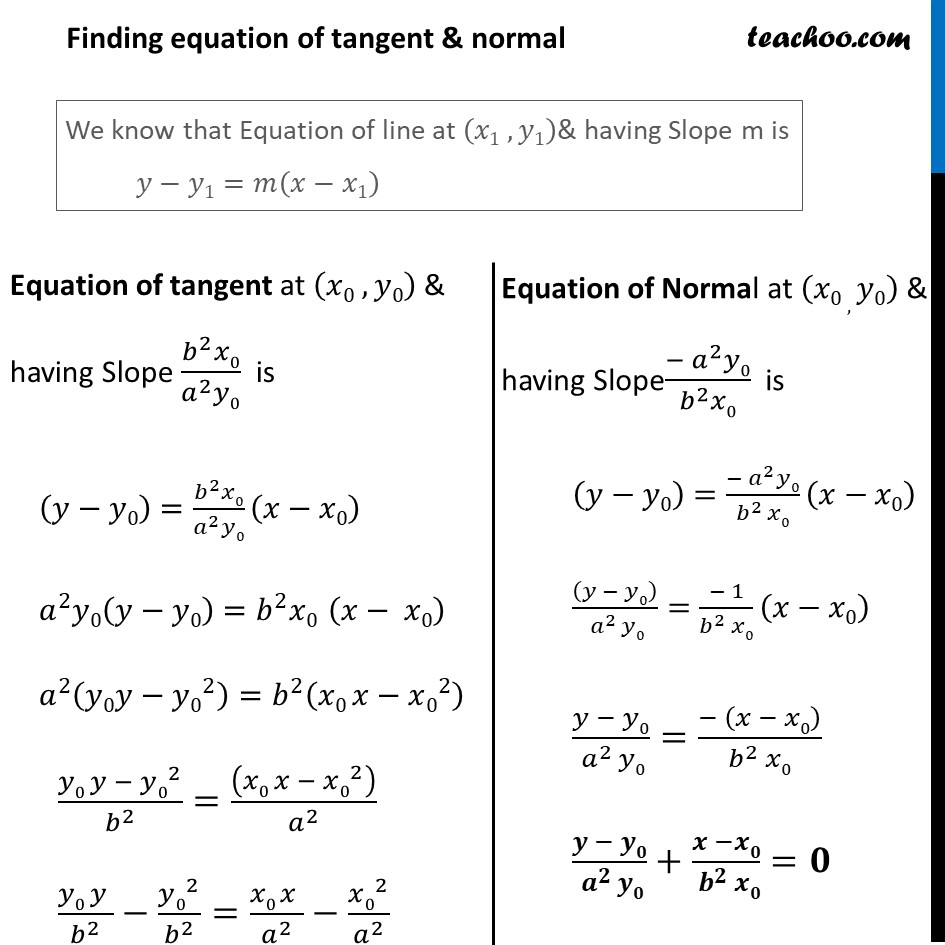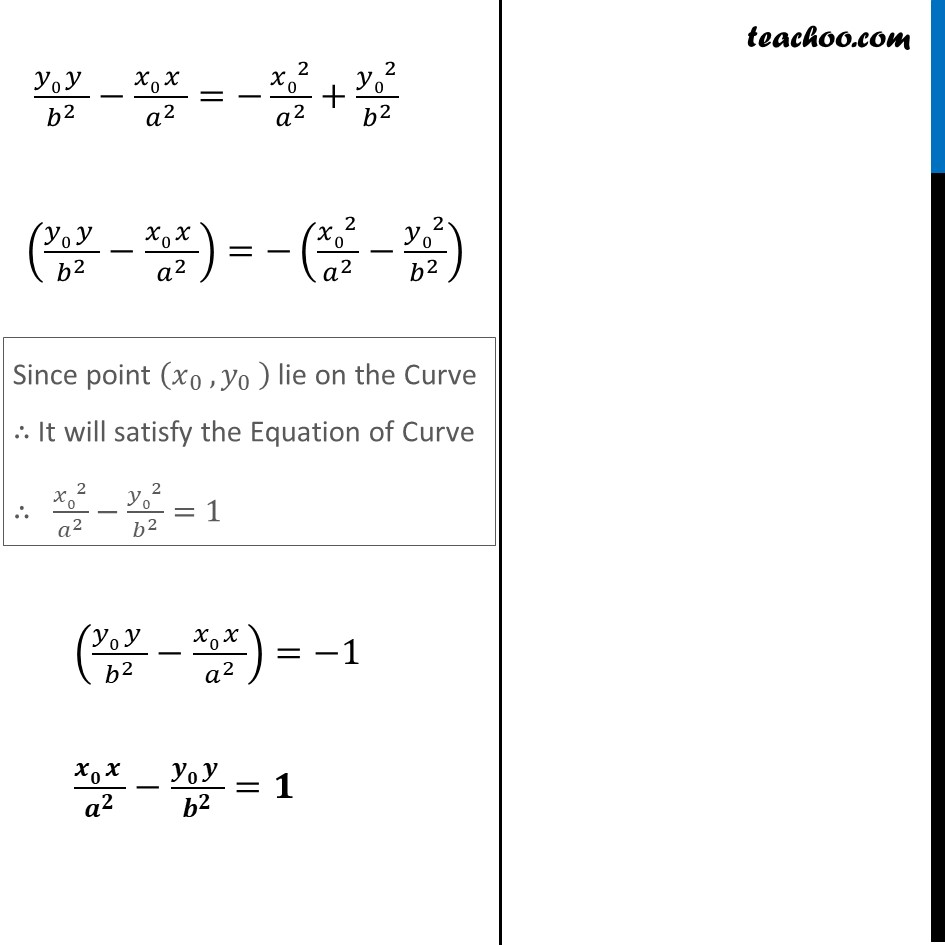Learn in your speed, with individual attention - Teachoo Maths 1-on-1 Class

### Transcript

Question 24 Find the equations of the tangent and normal to the hyperbola 𝑥^2/𝑎^2 – 𝑦^2/𝑏^2 = 1 at the point (𝑥0 , 𝑦0)We know that Slope of tangent is 𝑑𝑦/𝑑𝑥 Finding 𝒅𝒚/𝒅𝒙 𝑥^2/𝑎^2 −𝑦^2/𝑏^2 =1 −𝑦^2/𝑏^2 =1−𝑥^2/𝑎^2 𝑦^2/𝑏^2 =𝑥^2/𝑎^2 −1 Differentiating w.r.t.𝑥 𝑑(𝑦^2/𝑏^2 )/𝑑𝑥=𝑑(𝑥^2/𝑎^2 −1)/𝑑𝑥 1/𝑏^2 𝑑(𝑦^2 )/𝑑𝑥=𝑑/𝑑𝑥 (𝑥^2/𝑎^2 )−𝑑(1)/𝑑𝑥 1/𝑏^2 × 𝑑(𝑦^2 )/𝑑𝑥 × 𝑑𝑦/𝑑𝑦=1/𝑎^2 𝑑(𝑥^2 )/𝑑𝑥−0 1/𝑏^2 𝑑(𝑦^2 )/𝑑𝑦 × 𝑑𝑦/𝑑𝑥=1/𝑎^2 . 2𝑥 1/𝑏^2 ×2𝑦 × 𝑑𝑦/𝑑𝑥=1/𝑎^2 2𝑥 𝑑𝑦/𝑑𝑥=2𝑥/𝑎^2 × 𝑏^2/2𝑦 𝑑𝑦/𝑑𝑥=(𝑏^2 𝑥)/(𝑎^2 𝑦) Slope of tangent at (𝑥0 , 𝑦0) is 〖𝑑𝑦/𝑑𝑥│〗_((𝑥0 , 𝑦0) )=(𝑏^2 𝑥0)/(𝑎^2 𝑦0) We know that Slope of tangent × Slope of Normal =−1 (𝑏^2 𝑥0)/(𝑎^2 𝑦0) × Slope of Normal =−1 Slope of Normal = (−〖 𝑎〗^2 𝑦0)/(𝑏^2 𝑥0) 𝑑𝑦/𝑑𝑥=(𝑏^2 𝑥)/(𝑎^2 𝑦) Slope of tangent at (𝑥0 , 𝑦0) is 〖𝑑𝑦/𝑑𝑥│〗_((𝑥0 , 𝑦0) )=(𝑏^2 𝑥0)/(𝑎^2 𝑦0) We know that Slope of tangent × Slope of Normal =−1 (𝑏^2 𝑥0)/(𝑎^2 𝑦0) × Slope of Normal =−1 Slope of Normal = (−〖 𝑎〗^2 𝑦0)/(𝑏^2 𝑥0) Finding equation of tangent & normal We know that Equation of line at (𝑥1 , 𝑦1)& having Slope m is 𝑦−𝑦1=𝑚(𝑥−𝑥1) Equation of tangent at (𝑥0 , 𝑦0) & having Slope (𝑏^2 𝑥0)/(𝑎^2 𝑦0) is (𝑦−𝑦0)=(𝑏^2 𝑥0)/(𝑎^2 𝑦0) (𝑥−𝑥0) 𝑎^2 𝑦0(𝑦−𝑦0)=𝑏^2 𝑥0 (𝑥− 𝑥0) 𝑎^2 (𝑦0𝑦−𝑦0^2 )=𝑏^2 (𝑥0 𝑥−𝑥0^2 ) (𝑦0 𝑦 − 𝑦0^2)/𝑏^2 =((𝑥0 𝑥 − 𝑥0^2 ))/𝑎^2 (𝑦0 𝑦 )/𝑏^2 −(𝑦0^2)/𝑏^2 =(𝑥0 𝑥 )/𝑎^2 −(𝑥0^2)/𝑎^2 Equation of Normal at (𝑥0 ,𝑦0) & having Slope(−〖 𝑎〗^2 𝑦0)/(𝑏^2 𝑥0) is (𝑦−𝑦0)=(−〖 𝑎〗^2 𝑦0)/(𝑏^(2 ) 𝑥0) (𝑥−𝑥0) ((𝑦 − 𝑦0))/(〖 𝑎〗^(2 ) 𝑦0)=(− 1)/(𝑏^(2 ) 𝑥0) (𝑥−𝑥0) (𝑦 − 𝑦0)/(〖 𝑎〗^(2 ) 𝑦0)=(− (𝑥 − 𝑥0))/(𝑏^(2 ) 𝑥0) (𝒚 − 𝒚𝟎)/(〖 𝒂〗^(𝟐 ) 𝒚𝟎)+(𝒙 −𝒙𝟎)/(〖 𝒃〗^(𝟐 ) 𝒙𝟎)=𝟎 (𝑦0 𝑦 )/𝑏^2 −(𝑥0 𝑥 )/𝑎^2 =−(𝑥0^2)/𝑎^2 +(𝑦0^2)/𝑏^2 ((𝑦0 𝑦 )/𝑏^2 −(𝑥0 𝑥 )/𝑎^2 )=−((𝑥0^2)/𝑎^2 −(𝑦0^2)/𝑏^2 ) ((𝑦0 𝑦 )/𝑏^2 −(𝑥0 𝑥 )/𝑎^2 )=−1 (𝒙𝟎 𝒙 )/𝒂^𝟐 −(𝒚𝟎 𝒚 )/𝒃^𝟐 =𝟏 Since point (𝑥_0 ,𝑦_0 ) lie on the Curve ∴ It will satisfy the Equation of Curve ∴ (𝑥0^2)/𝑎^2 −(𝑦0^2)/𝑏^2 =1